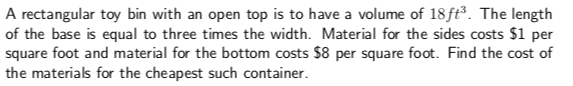A rectangular toy bin with an open top is to have a volume of 18ft3. The lengthof the base is equal to three times the width. Material for the sides costs \$1 persquare foot and material for the bottom costs \$8 per square foot. Find the cost ofthe materials for the cheapest such container

Questionhelp_outlineImage TranscriptioncloseA rectangular toy bin with an open top is to have a volume of 18ft3. The length of the base is equal to three times the width. Material for the sides costs \$1 per square foot and material for the bottom costs \$8 per square foot. Find the cost of the materials for the cheapest such container fullscreen
Step 1

The volume of the rectangular toy bin with an open top is 18 ft3. Let the dimension of the bin be x ft by y ft by z ft. Then the equation becomes

Step 2

The length of the base is equal to three times the width that is

Step 3

Substitute x in xyz = 18 and o...

Want to see the full answer?

See Solution

Want to see this answer and more?

Our solutions are written by experts, many with advanced degrees, and available 24/7

See Solution
Tagged in

Derivative